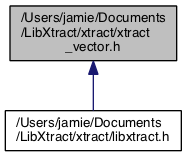LibXtract  0.7.1
xtract_vector.h File Reference
This graph shows which files directly or indirectly include this file:Go to the source code of this file.

Functions

int xtract_spectrum (const double *data, const int N, const void *argv, double *result)
Extract frequency domain spectrum from time domain signal. More...

int xtract_autocorrelation_fft (const double *data, const int N, const void *argv, double *result)
Extract autocorrelation from time domain signal using FFT based method. More...

int xtract_mfcc (const double *data, const int N, const void *argv, double *result)
Extract Mel Frequency Cepstral Coefficients based on a method described by Rabiner. More...

int xtract_dct (const double *data, const int N, const void *argv, double *result)
Extract the Discrete Cosine transform of a time domain signal. More...

int xtract_autocorrelation (const double *data, const int N, const void *argv, double *result)
Extract autocorrelation from time domain signal using time-domain autocorrelation technique. More...

int xtract_amdf (const double *data, const int N, const void *argv, double *result)
Extract Average Magnitude Difference Function from time domain signal. More...

int xtract_asdf (const double *data, const int N, const void *argv, double *result)
Extract Average Squared Difference Function from time domain signal. More...

int xtract_bark_coefficients (const double *data, const int N, const void *argv, double *result)
Extract Bark band coefficients based on a method. More...

int xtract_peak_spectrum (const double *data, const int N, const void *argv, double *result)
Extract the amplitude and frequency of spectral peaks from a magnitude spectrum. More...

int xtract_harmonic_spectrum (const double *data, const int N, const void *argv, double *result)
Extract the harmonic spectrum of from a of a peak spectrum. More...

int xtract_lpc (const double *data, const int N, const void *argv, double *result)
Extract Linear Predictive Coding Coefficients. More...

int xtract_lpcc (const double *data, const int N, const void *argv, double *result)
Extract Linear Predictive Coding Cepstral Coefficients. More...

int xtract_subbands (const double *data, const int N, const void *argv, double *result)
Extract subbands from a spectrum. More...

Detailed Description

: declares functions that extract a feature as a vector from an input vector